Custom SearchThere is a large variety of terminations for rf lines. Each type of termination has a characteristic effect on the standing waves on the line. From the nature of the standing waves, you can determine the type of termination that produces the waves. TERMINATION IN Z0 Termination in Z0 (characteristic impedance) will cause a constant reading on an ac meter when it is moved along the length of the line. As illustrated in figure 3-34, view A, the curve, provided there are no losses in the line, will be a straight line. If there are losses in the line, the amplitude of the voltage and current will diminish as they move down the line (view B). The losses are due to dc resistance in the line itself. Figure 3-34. - Effects of various terminations on standing waves.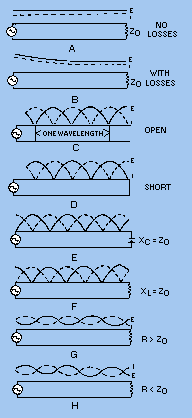In an open-circuited rf line (figure 3-34, view C), the voltage is maximum at the end, but the current is minimum. The distance between two adjacent zero current points is 1/2l, and the distance between alternate zero current points is 1l. The voltage is zero at a distance of 1/4l from the end of the line. This is true at any frequency. A voltage peak occurs at the end of the line, at 1/2l from the end, and at each 1/2l thereafter. TERMINATION IN A SHORT CIRCUIT On the line terminated in a short circuit, shown in figure 3-34, view D, the voltage is zero at the end and maximum at 1/4l from the end. The current is maximum at the end, zero at 1/4l from the end, and alternately maximum and zero every 1/4l thereafter. TERMINATION IN CAPACITANCE When a line is terminated in capacitance, the capacitor does not absorb energy, but returns all of the energy to the circuit. This means there is 100 percent reflection. The current and voltage relationships are somewhat more involved than in previous types of termination. For this explanation, assume that the capacitive reactance is equal to the Z0 of the line. Current and voltage are in phase when they arrive at the end of the line, but in flowing through the capacitor and the characteristic impedance (Z0) connected in series, they shift in phase relationship. Current and voltage arrive in phase and leave out of phase. This results in the standing-wave configuration shown in figure 3-34, view E. The standing wave of voltage is minimum at a distance of exactly 1/8l from the end. If the capacitive reactance is greater than Z0 (smaller capacitance), the termination looks more like an open circuit; the voltage minimum moves away from the end. If the capacitive reactance is smaller than Z0, the minimum moves toward the end. TERMINATION IN INDUCTANCE When the line is terminated in an inductance, both the current and voltage shift in phase as they arrive at the end of the line. When XL is equal to Z0, the resulting standing waves are as shown in figure 3-34, view F. The current minimum is located 1/8l from the end of the line. When the inductive reactance is increased, the standing waves appear closer to the end. When the inductive reactance is decreased, the standing waves move away from the end of the line. TERMINATION IN A RESISTANCE NOT EQUAL TO THE CHARACTERISTIC IMPEDANCE (Z0) Whenever the termination is not equal to Z0, reflections occur on the line. For example, if the terminating element contains resistance, it absorbs some energy, but if the resistive element does not equal the Z0 of the line, some of the energy is reflected. The amount of voltage reflected may be found by using the equation: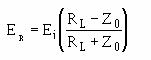Where: ER = the reflected voltage Ei = the incident voltage RR = the terminating resistance Z0= the characteristic impedance of the line If you try different values of RL in the preceding equation, you will find that the reflected voltage is equal to the incident voltage only when RL equals 0 or is infinitely large. When RLequals Z0, no reflected voltage occurs. When RLis greater than Z0, ER is positive, but less than Ei. As RL increases and approaches an infinite value, ER increases and approaches Ei in value. When RL is smaller than Z0, ER has a negative value. This means that the reflected voltage is of opposite polarity to the incident wave at the termination of the line. As RL approaches zero, ER approaches Ei in value. The smaller the value of ER, the smaller is the peak amplitude of the standing waves and the higher are the minimum values. TERMINATION IN A RESISTANCE GREATER THAN Z0 When RL is greater than Z0, the end of the line is somewhat like an open circuit; that is, standing waves appear on the line. The voltage maximum appears at the end of the line and also at half-wave intervals back from the end. The current is minimum (not zero) at the end of the line and maximum at the odd quarter-wave points. Since part of the power in the incident wave is consumed by the load resistance, the minimum voltage and current are less than for the standing waves on an open-ended line. Figure 3-34, view G, illustrates the standing waves for this condition. TERMINATION IN A RESISTANCE LESS THAN Z0 When RL is less than Z0, the termination appears as a short circuit. The standing waves are shown in figure 3-34, view H. Notice that the line terminates in a current LOOP (peak) and a voltage NODE (minimum). The values of the maximum and minimum voltage and current approach those for a shorted line as the value of RL approaches zero. A line does not have to be any particular length to produce standing waves; however, it cannot be an infinite line. Voltage and current must be reflected to produce standing waves. For reflection to occur, a line must not be terminated in its characteristic impedance. Reflection occurs on lines terminated in opens, shorts, capacitances, and inductances, because no energy is absorbed by the load. If the line is terminated in a resistance not equal to the characteristic impedance of the line, some energy will be absorbed and the rest will be reflected. The voltage and current relationships for open-ended and shorted lines are opposite to each other, as shown in figure 3-34, views C and D. The points of maximum and minimum voltage and current are determined from the output end of the line, because reflection always begins at that end. Q.26 A nonresonant line is a line that has no standing waves of current and voltage on it and is considered to be flat. Why is this true?Q.27 On an open line, the voltage and impedance are maximum at what points on the line?STANDING-WAVE RATIO The measurement of standing waves on a transmission line yields information about equipment operating conditions. Maximum power is absorbed by the load when ZL = Z0. If a line has no standing waves, the termination for that line is correct and maximum power transfer takes place. You have probably noticed that the variation of standing waves shows how near the rf line is to being terminated in Z0. A wide variation in voltage along the length means a termination far from Z0. A small variation means termination near Z0. Therefore, the ratio of the maximum to the minimum is a measure of the perfection of the termination of a line. This ratio is called the STANDING-WAVE RATIO (swr) and is always expressed in whole numbers. For example, a ratio of 1:1 describes a line terminated in its characteristic impedance (Z0). Voltage Standing-Wave Ratio The ratio of maximum voltage to minimum voltage on a line is called the VOLTAGE STANDING-WAVE RATIO (vswr). Therefore: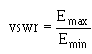The vertical lines in the formula indicate that the enclosed quantities are absolute and that the two values are taken without regard to polarity. Depending on the nature of the standing waves, the numerical value of vswr ranges from a value of 1 (ZL = Z0, no standing waves) to an infinite value for theoretically complete reflection. Since there is always a small loss on a line, the minimum voltage is never zero and the vswr is always some finite value. However, if the vswr is to be a useful quantity, the power losses along the line must be small in comparison to the transmitted power. Power Standing-Wave Ratio The square of the voltage standing-wave ratio is called the POWER STANDING-WAVE RATIO (pswr). Therefore: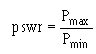This ratio is useful because the instruments used to detect standing waves react to the square of the voltage. Since power is proportional to the square of the voltage, the ratio of the square of the maximum and minimum voltages is called the power standing-wave ratio. In a sense, the name is misleading because the power along a transmission line does not vary. Current Standing-Wave Ratio The ratio of maximum to minimum current along a transmission line is called CURRENT STANDING-WAVE RATIO (iswr). Therefore: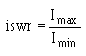This ratio is the same as that for voltages. It can be used where measurements are made with loops that sample the magnetic field along a line. It gives the same results as vswr measurements. Q.28 At what point on an open-circuited rf line do voltage peaks occur?Q.29 What is the square of the voltage standing-wave ratio called?Q.30 What does vswr measure?Integrated Publishing, Inc. - A (SDVOSB) Service Disabled Veteran Owned Small Business